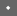COOTS 1998    [Technical Program]

 Pp. 249–264 of the ProceedingsCompile Time Symbolic Derivation with C++ Templates

 Joseph (Yossi) Gil IBM T.J. Watson Research Center P.O. Box 704 Yorktown Heights, NY 10598 Zvi Gutterman Faculty of Computer Science Technion Israel Institute of Technology Haifa 32000, Israel

1. Abstract

C++ templates are already recognized as a powerful linguistic mechanism, whose usefulness transcends the realization of traditional generic containers. In the same venue, this paper reports on a somewhat surprising application of templates—for computing the symbolic derivative of expression. Specifically, we describe a software package based on templates, called SEMT, which allows the programmer to create symbolic expressions, substitute variables in them, and compute their derivatives. SEMT is unique in that these manipulations are all done at compile time. In other words, SEMT effectively coerces the compiler to do symbolic computation as part of the compilation process. Beyond the theoretical interest, SEMT can be practically applied in the efficient, generic and easy to use implementation of many numerical algorithms.

KEYWORDS: SCIENTIFIC COMPUTING, GENERIC PROGRAMMING, NUMERICAL ALGORITHMS, SYMBOLIC DERIVATION.

1. Introduction
2. C++ templates were originally designed to support the realization of traditional generic containers and algorithms [S94: Chap. 15]. However, there is much more to C++ templates beyond basic genericity capabilities: function templates and their implicit instantiation mechanism and other sophisticated specialization mechanisms, a variety of kinds of parameters, including parameters which are template themselves, default parameters, explicit instantiation, and more. C++ genericity mechanism also draws power from the fact that a class template is not limited to methods and instance variables. It may include static member data members; type and constant definitions (using typedef and enum), nested classes and even nested template classes and template function members. The combined power of all these enable a relatively new language feature to surpass its original intent. A well known (and at first sight intriguing) example of this is that of function objects and binders [KM96: Chap. 21-22] which are part of the Standard Template Library (STL) [MS96]. Another interesting use of templates is that of "trait classes" [NM95] which were employed in dealing with the internationalization of C++ code.

The work reported here is an extension of this line of inquiry. We demonstrate the practical implementation of C++ templates in doing some non-trivial symbolic computation during compile time. To a certain extent we continue the work on template metaprograms technique of Veldhuizen [V95a] in which the compiler is employed as an interpreter. The instructions to this interpreter are encoded in an elaborate template lingo. The useful work that this interpreter does is the generation of efficient inlined code. Template metaprograms were exemplified in making the compiler evaluate the factorial function instead of deferring its computation to run-time. Yet another application of these was the efficient computation of a prefix of a Taylor series expansion for approximating numerical functions.

The template metaprograms technique was perfected in Veldhuizen's subsequent work [V95b] on expression templates. Expression templates were used to create an efficient inlined code for expressions on vectors and matrices. Consider the C++ expression, A+B*c, where A and B are vectors of the same length, and c is some scalar. In a simple-minded operator overloading implementation, this expression would span two loops. However, using expression templates for it effects a code that comprises a single loop, as it would have been by manually crafted code.

Our extension to expression templates is a template based software package called SEMT ("Symbolic Expressions Manipulation with Templates"). SEMT is different than the work of [V95b] in that the symbolic expressions can be directly created, used and manipulated by the programmer, rather than just serving as internal representation employed in the optimization purpose. One important and far from trivial such manipulation is the computation of the symbolic derivative. Again, the use of templates makes it possible to do these manipulations at compile time. In other words, SEMT effectively coerces the compiler to do symbolic computation as part of the compilation process. Beyond the theoretical interest, SEMT is applicable in an efficient, generic and easy to use implementation of the many numerical algorithms that make use of the derivative function. A primary example of such an algorithm is the Newton-Raphson method for finding the roots of a function.

Outline. The rest of this paper is structured as follows. The next Section 2 makes the case for symbolic manipulations in compile time. In Section 3 we explain the technique of using types to represent expressions. Section 4 describes symbolic derivation and computing the value of an expression at a given numerical point. The implementation and evaluation of the run time performance of the package are the subject of Section 5. The penultimate Section 6 presents some more sophisticated applications employing more advanced techniques. The technical discussion also culminates here in implementing the chain rule using C++ templates. Finally, Section 7 draws the conclusions and lays out some directions for future research.

3. The Case for Symbolic,
Compile-Time Derivation
4. A familiar example of the use of the derivative function in scientific computing is that of the Newton-Raphson method. This method, which will be used as our main running example, prescribes that, the next iterative approximation to a root of a function, is computed from the previous approximation, using the following formula:Although the method is simple in principle, its implementation will necessarily depend on many parameters: an initial guess, bounds on the accepted final error (e.g., asor), an upper limit to the number of iterations, the functionitself, the algebraic field (real vs. complex), the arithmetic precision with which the computation is carried out, , etc. Such dependencies are not a phenomenon specific to Newton-Raphson or to numerical computation only. Software of all kinds exhibits dependency on a large number of widely different parameters. In general, a dependency on a certain parameter can be captured at one of the following three points during a program lifetime.

1. Run Time. An implementation may receive a setting for the parameter at run time as an actual parameter of a routine or as a global variable. For an example, consider the implementation of Newton-Raphson algorithm in Figure  1 below. The initial guess and an error bound onare formal parameters to function NR_simple; their values are determined only when routine NR_simple starts its run.

The run-time dependency approach is the most flexible, since the same compiled code can be used for all settings of the parameter. This approach is also the least efficient, since the code must consult the parameter value during its execution. Furthermore, this approach is more difficult to optimize in a separate compilation environment.

2. Compile Time. In this approach to the expression of the dependency on a parameter, the same source is compiled to different object targets, depending on the parameter value. In Figure  1, identifier MAX_ITERATIONS is a pre-processor constant, to be set at compile time by the programmer. A different object code will be generated for each different setting of it.

This approach incurs no direct performance penalty, although the coding for generality to account for different parameter values may bring about an implicit cost. Compile time parameters are also safer than run-time parameters, since the compiler gets a chance to check the parameters. Erroneous parameters would lead to a compilation-, rather than a run-time error.

3. Design Time. In this approach, the human programmer writes the code for a specific value of the parameter. In Figure  1, for example, it was a design decision to use double precision arithmetic. When the need arises to change the value of the parameter, the human software engineer is called in to write a new code, or rewrite the existing one.

In most cases, the intermediate approach of compile-time parameter dependency is the preferred one. Compile-time parameters make it possible to achieve flexibility without compromising efficiency. The cost is in the coding effort and sometimes in portability to other languages and environments. Implementing compile-time parameters is typically not easy. Such implementation is often highly dependent on the expressive power of the underlying programming language. Standard C environment, for example, does not directly support simultaneous different object files of the same source code. It takes proficiency in writing makefile and skills in juggling pre-processor directives to convert the code in Figure  1 to make the arithmetical precision design-time parameter into a compile-time one. It is even more challenging to do the same for the function run-time parameter.

C++ templates open the door to a new world of cleaner representation of compile-time parameters. It is straightforward to make precision a compile-time parameter. But, in general, template programming is much more difficult. In order to avoid the cost of indirect function call one may want to make function and tag_function into true compile-time parameters. But this can only be done using the sophisticated function-objects technique of STL. The function objects technique suggests that there is more to template programming than meets the eye. Another indication of this is the clever use of templates to express policy parameters, such as memory allocation schemes, algorithmic strategies, etc. (See e.g., [MS96] and [S91: Chap. 13.4].) In this broader context we may regard the contribution of this paper as a further exploration of the expressive power of C++ templates. We demonstrate that a non-trivial symbolic computation can be carried out at compile time using templates.

In the more specific Newton-Raphson example, there is a design-time dependency of the derivative function tag_function on function. For each value of function, the programmer must code in a new derivative to be passed as parameter to tag_function. Thus, to solve the equation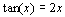the programmer would not only have to code the following function

double G(double x) {
return tan(x) - 2 * x;

}

but also,

double G_tag(double x) {
return 1.0/pow(cos(x),2) - 2;

}

only after which a call to the numeric solver such as

cout << "The root of Tan(x) is " <<
NR_simple(G, G_tag, 1.0, 1E-5);

can be issued.

The code of function G_tag depends on that of function G. Whenever G changes so should G_tag. This dependency was captured at design time: whenever G changes, the programmer must be called in to recode G_tag. The run-time approach alternative to this is to compute, or rather approximate, the derivative at run-time. However, the cost of doing so would never be acceptable in a fast numeric solver.

Using SEMT, the dependency ofoncan be shifted from design time into compile-time one. The user may write a functionmuch like any C++ expression, and reply on the compiler to symbolically compute expression. These expressions are implemented as C++ types. A call to the SEMT solver might take the following form:TYPE(tan(x) - 2*x) g;
double x = 0.98;
cout << "The root of " << g
<< " near " << x << " is "
<< NR_symbolic(g, 1.0, 1E-5);

which would produce the output

The root of Tan(X) - 2 * X near 0.98 is 1.1656

The macro TYPE returns the type of an expression. It is easy enough to implement in gcc,Gnu’s C++ compiler, with the use of the typeof operator. Unfortunately, as far as we could determine, it is impossible to implement in a standard-conforming compiler. With C++ implementations that do not support this language extension we must resort to a more complicated scheme to extract the type of an expression, in lieu of the elegant typeof operator and the TYPE macro. This scheme is discussed below.

Note how a symbolic expression can be stored in a type of a variable g. Passing the variable as a parameter is the same as passing the type associated with it as a parameter. Evidently, the syntax for invoking NR_symbolic is cleaner. Also, the code is less bug-prone, since the user is no longer trusted (or troubled) to carry out the symbolic derivation by hand and keep it in synchronization with the original function. From an efficiency perspective, bothandcould be inlined into the numeric solver, thus achieving the same level of specialization as that of the hand-coded solver of Figure  2.

5. Expressions as Types

In this section we describe the basic idea behind used in SEMT, which is similar in principle to that of [V95b]. It is to have a distinct C++ class, generated by a template, for each possible symbolic expression. We start by giving a more formal definition of the symbolic expression concept:

Definition 1 The setof all symbolic expressions is defined as the minimal set such that:

1. all integer constantsare in;
2. symbolic variables,are in;
3. for each unary operator, and for each function,if, then so areand; and ,
4. for each binary operator, ifthen so is.

Ignoring momentarily the fact that non-class types (such as int and ios *) may serve as template parameters, we can think of class templates with typename parameters as functions, operating at compile time, whose domain and range is the universe of all classes. Equating this with the above definition, we can devise a recursive construction of a C++ class for each symbolic expression:

1. To create a distinct class for each such integer value, there is a class template expecting a constant int parameter.
2. There are unique X, Y,… C++ classes to represent the symbolic variables,. Alternatively, there could be a template expecting a char constant argument, such as 'x', 'y', …, as a parameters to create a unique class for the symbolic variables.
3. For each unary operator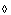and for each function, there is a unique class template that takes the class corresponding to expressionas a parameter and creates a new class forand for.
4. For each binary operatorthere is a class template that takes the classes corresponding to symbolic expressionsand, and creates a new class that corresponds to.

In this construction, there be would an instance of class template sin_t (which takes a single typename parameter) to represent an expression of the form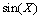. There would be an instance of the class template plus_t to represent an expression whose outer most operation is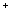. Also, there would a unique class to represent each symbolic variable used in the program, and a template class, instantiated out of a class template taking a constant int parameter, for each integer constant used as part of a symbolic expression in the program. The following C++ code excerpt demonstrates the idea:

template<typename T> class sin_t {};
template<typename T1, typename T2>
class plus_t {};

// ...

template<int n> class Number {};
class Variable {};

With this, the symbolic expressionis represented as the template class plus_t<Variable, sin_t< plus_t< Variable,sin_t<Variable> > > >, or in somewhat easier to read indented layout:

plus_t<
Variable,
sin_t<
plus_t<
Variable,
sin_t<Variable>
>
>
>

We see that a type definition can be very long and unwieldy even for a relatively small expression. We cannot improve on this in the general case, syntactic sugaring is possible for many applications by using function templates such as:

template<typename T>
inline sin_t<T> sin(const T& p) {
return sin_t<T>();
}

Template function sin returns a value of type sin_t<> for any type. More importantly, thanks to the implicit template instantiation, the return type of sin(v) for a value v of type e would be sin_t<e *>. Thus, instead of having a type corresponding to a symbolic expression, we have a value of the exact same type, which is returned by that function. Note how simple the body of the function is. It can be further simplified using a pointer return type to eliminate the constructor call from the return statement:

template<typename T>
inline const sin_t<T> * const sin(
const T * const) {
return 0;
}

template<typename T1, typename T2>
inline const plus_t<T1,T2> * const
operator+(
const T1 *const,
const T2 *const) {
return 0;
}

Now, X + sin(X + sin(X)) is a C++ expression whose type is a (const) pointer to (a const of) the type corresponding to expression. Some non-trivial compiler work needs to be done to discover that type. However, in evaluating this expression, there is little, if any code generation required of the compiler. Even a half-hearted optimization attempt of this C++ expression will quickly reveal that it is nothing but the null pointer.

We use the term expression values for null pointers of this kind. Also, template functions, such as the two defined above, which take and return expression values without doing any computation on them, are called expression functions. The following macro definition is useful for defining expression functions:

#define E_VALUE(T) const T * const

The basic expression functions for type expressions can be concisely coded using two more simple macros as shown in Figure  3

1. Compositional Recursion
2. In this section we describe how symbolic derivation is implemented in SEMT. The exposition is served by starting with a sketch of the implementation of expression application: the process by which value of an expression at a certain numerical point is evaluated. We then proceed to describe symbolic derivation. Figure  4 demonstrates how these two operations might be used in actual user code. As before, the C++ variables u and v are dummies. Their actual value is less important than their type. Thus, the type of u represents the constant 10, while type of v represents a free variable.

Function apply is a template function which is instantiated from the following function template.

template <typename T>
inline double apply(
E_VALUE(T),
double val) {
return T::apply(val);
}

(It is also possible to make the arithmetical precision a parameter of the template, but for simplicity, we will just use double.) The type of the first parameter represents the symbolic expression. The instantiation of the function template depends on this type. The second parameter of apply is the point where that expression has to be evaluated.

The function template apply works since each C++ class which corresponds to a symbolic expression would have a static function apply, defined by what we will call compositional recursion on the type, or equivalently, the expression structure, to do the actual evaluation. Figure  5 illustrates the appropriate class template definitions for making the compositional recursion definition of apply.

The recursion base is classes Variable and Number representing variable and constants in the expression. For the sake of brevity, only the templates for the unaryand the binaryexpression type constructors are shown in this and the other code excerpts that follow.

Note that compositional recursion is somewhat reminiscent of refinement in inheritance, in which an overriding function relies on the implementation of the overridden one for its own implementation.

In much the same way, one can define a print function (or an operator<<) to print the symbolic expression that each class represents. We do not include the code for this here.

Compositional recursion is basically the technique of implementing the derivative operator. However, since symbolic derivation is a mapping from symbolic expressions to symbolic expressions, it should be represented as a class to class mapping. For this, we must rely on the ability to make typedef declarations in a class. The following typedef makes the derivative of a free variable to be the constant 1

class Variable {
public:
typedef Number<1> tag;
};

The actual code is depicted in Figure  6. For brevity, only the tag typedef members of the class templates are shown in the Figure ; we will remain faithful to this convention of illustrating only the new members of previously defined classes.

For each type T representing a symbolic expression, type T::tag represents the type of the derivative of the symbolic expression that T represents. At this stage, we do not distinguish between different symbolic variables that might occur in type T. For the purpose of derivation and application, all symbolic variables are considered identical. Thus, all symbolic expressions can be thought of as functions of one variable.

Here is the definition of function derive which makes it possible to apply symbolic derivations not only to types, but also to expression values.

template <typename T>
E_VALUE(T::tag) derive(E_VALUE(T)) {
return 0;
}

3. Implementation and Performance
4. The source code of SEMT spans some twelve hundred lines of C++ code, which are to be put on the web shortly. SEMT supports the elementary mathematical operators as well as standard mathematical functions including the trigonometric functions and their inverse and logarithm and exponentiation. Unfortunately, it has been difficult to get the entire code to compile on most current compilers although different pieces have compiled correctly on different compilers. This includes also the leading three compilers (Borland, Gnu and Microsoft). It is clear that current compilers are still lacking in providing full support of the upcoming ISO/ANSI C++ standard [IA97]. Some of the weaknesses that we have encountered are support for nested template classes, template specialization and template functions with non-typename arguments.

SEMT does not yet support arithmetical precision as a template parameter, but work on this extension has already begun.

Due to the highly structured nature of the definitions, most of the code can be written using sophisticated macros, similar to those of Figure  3. They have been found essential to capture the commonality between many template definitions.

As a side comment we note that extensive use of pre-processor macros is often frowned upon in ordinary C++ programming. Many of their traditional applications in C are better served with the more modern features of C++. Templates were promoted and effectively used as a structured substitute to macros. It is therefore especially displeasing to have to resort to macros in a template package. We find this need to be the result of lack of higher level constructs in C++. There are no templates for defining templates, templates for defining templates defining templates, etc. The sophisticated recent additions to the language, such as passing a template argument to a template do not seem to ameliorate this predicament.

The implementation of the Newton-Raphson algorithm as an expression function in SEMT is given in Figure  7, which is arguably more elegant than that of Figure  1, but potentially as efficient as the hand-coded, specialized version of Figure  2.

Table 1 compares the running time of an implementation of the Newton-Raphson algorithm which is template-based Figure  7 to one which uses pointers to functions (Figure  1) for several functions of varying level of complexity. The Visual C++ compiler (Version 5.0) generated the code. Measurements were taken on a 32MB, 133MHZ Pentium machine.

It can be inferred from the table that in finding the roots of relatively simple functions, the template version does not significantly improve the run time of the algorithm in comparison to the standard implementation. However, for more complex functions, the differences in run time become more substantial. These observations are consistent with our conjecture that in small functions, the main saving is due to the elimination of indirect function call. More complicated expressions give rise to more optimization opportunities due to code inlining in the template based implementation.

We do not ignore the fact that straightforward symbolic derivation may produce gigantic expressions which may greatly benefit from a substantial symbolic simplification which a compiler is unlikely to make. (Consider for example the 17th derivative of. A naïve derivation would yield 217 terms, which can be simplified to only 18 terms.) In a template-based implementation, an optimizing compiler is given a fair chance to run massive code transformations, which may produce equivalent results. Curiously, this offers a perspective of engaging the optimizer module of the compiler in the task of symbolic simplification, a chore that is usually done manually, or with the aid of a dedicated package. From our limited experiments, it is not clear to what extent current compilers are capable in this task.

 Function Pointers Templates1415 1408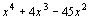2162 1590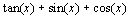3224 24274223 28964500 3510

5. Higher Applications and Advanced Techniques
6. In this section we describe how the ideas behind symbolic derivation described above can be used for doing a variety of symbolic manipulations at compile time. These include rational arithmetic, substitution (superimposition), taking the nth derivative, partial derivation and the chain rule.

1. Rational Arithmetic
2. Unfortunately, floating point numbers cannot serve as parameters to C++ templates. Thus, constants used in our symbolic expressions were all of type integer. This is less of a limitation than it may seem, since real numbers can be approximated with rational expression such as Number<31>/Number<7>. Moreover, note that symbolic expressions involving only constants offer unbounded precision. The value type

1/(1 + 1/(1 +
1/(1 + 1 / (1 + 1/…. + Number<1> )))…

gives an approximation to the golden ratio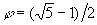which is arbitrarily close to it. However, when the need comes to evaluate an expression, the optimizing component of the compiler should be trusted to do the arithmetical simplifications. Alternatively, it is possible to use a rational arithmetic templates package that is structured much like the symbolic expressions. The essential parts of the code for this are given in Figure  8.

Note that it in the template implementation of rational it is all but explicitly guaranteed that the evaluations are all done in compile time. A C++ compiler is required by the language standard to instantiate a template exactly once for each distinct pair of parameter values. In particular, this must be the case even if an integer parameter is given as a compound constant expression.

3. Expression Superimposition
4. As explained above, a symbolic expressionwith one symbolic variablecan be also thought of as a function, or a lambda expression. As such, it can be applied to another expression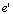to obtain the compound expression. We call this operation super-imposition. For example, super-imposingon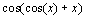would yield.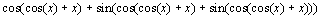Expression super-imposition is implemented using compositional recursion. This is done with help of a relatively new, and not widely supported, C++ feature known as nested template classes, or template class members. The code of this implementation is illustrated in Figure  9.

The following expression function helps to make the syntax of type superimposition simpler.

template <typename T, typename S>
E_VALUE( T::super<S>::impose* )
superimpose(
E_VALUE(T),
E_VALUE(S)) {
return 0;
}

Thus, the expression value for our example is created by:

superimpose(
X + sin(X + sin(X)),
cos(cos(X) + X)
)

5. N'th derivative
6. To compute derivatives of any arbitrary order, one can use the following code

template <typename T, int n>
class TAG_N { public:
typedef TAG_N<T::tag, n-1>::Tag tag;
};

template<typename T>
class TAG_N<T, 1> {
typedef T::tag tag;

};

In words, class template TAG_N taking parameters T and n, has a typedef tag which is the type corresponding to the nth derivative of the expression corresponding to type T. The definition of this typedef is made recursively: the nth derivative is the first derivative of the (n-1)th derivative. Template specialization for the case n=1 is the recursion base. An expression function to compute the arbitrary order derivatives is:

template <int n, typename T>
E_VALUE( TAG_N<T, n> )
n_derivative(E_VALUE<T>) {
return 0;
}

A call to this function to produce the expression value is:

n_derivative<3>(X + sin(X + sin(X)));

7. Partial Derivative

The code samples above used a single class, Variable, to represent symbolic variables in expression types. This means that expressions used only a single symbolic variable. All of the symbolic manipulations presented tacitly assumed that all variables in an expression are the same. Thus, typeof(X + 2 * Y - 3 * Z)::tag, where X and Y symbolic variables (i.e., instances of class Variable), is, (after some simplification)

Number<1> - Number<2> + Number<1>

which is nothing but 0. This is in contradiction with the basic rules of calculus which prescribe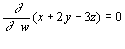It is easy enough to define a template to represent distinct symbolic variables. Here is one way of doing so:

template<char c> class Variable {
public:
// …

};

With this, template classes such as Variable<'x'> and Variable<'y'> serve as the symbolic variables.

In computing the partial derivative with respect to a specific variablethere are two issues that must be must be resolved. The first of these is that it is necessary to passdown the compositional recursion hierarchy. This is done using nested class templates as in Section 0 above. The other issue is the correct termination of the recursion in a class template Variable<x>, so that for a variable,, whereas. This is done using specialization of nested template classes, as illustrated in Figure  10.

An interesting application of partial derivatives is in the identityalso known in calculus as the "chain rule". An expression function to implement it is given in Figure  11:7. Conclusions and Further Research
8. We have described the main techniques behind SEMT—a C++ template package for manipulating symbolic expression at compile time. The package is useful in writing flexible and efficient code for numerical applications. The package can only be partially implemented on current compilers due to their lack of support of the standard. This is probably a temporary situation. We expect compilers to become more stable and standard conforming in the future. With this happening, a more closed implementation of the package will become available.

Many of the practical problems we experienced during the coding and porting were a result of the crass error messages and warnings that compilers issue for template expansion errors. The advent of advanced techniques of using C++ templates, of the sort propounded by this paper, builds a C++ code body which makes a sophisticated use of templates. This would hopefully coerce compiler writers to pay better attention to this point. Moreover, debugging code generated from templates is not easy. In many ways it resembles debugging high level code through the equivalent assembly. Again, improved tool support is required here.

The main C++ lingual feature that we feel is missing in order to make this package complete is a mechanism for extracting the type out of a value. This is required in order to translate an expression function back to the type of the value it returns, as done with the typeof pseudo-operator of Gnu C++ compiler.

We are currently engaged in extending the package to deal with algebraic numbers. Yet another interesting extension, with intriguing theoretical consequences, is to implement a full lambda calculus. On the other hand, the dual problem to the one we have solved here, namely coercing a symbolic computation such as Mathematica [W91] to compile C++ templates may be less than interesting.

Acknowledgments. Inspiring discussions with Irad Yavne and Mark Wegman are gratefully acknowledged.

9. References

[KM97] Koenig A., and B. Moo., Ruminations on C++, Addison Wesley 1996.

[IA97] ISO/Ansi, C++ Standard Draft Proposal X3J16, http://www.cygnus.com/misc/wp

[MS96] Musser, D. R., and A. Saini., STL– Tutorial and Reference Guide. Addison-Wesley, 1996.

[M95] Myers N., A new and useful template technique: "Traits", C++ Report 7(5):32–35, June 1995.

[TFP92] Teukolsky S. A., Flannery B. P., Press W. H., and W. T. Vetterling., Numerical recipes in C, Cambridge 1992.

[S91] Stroustrup B., The C++ Programming Language. 2nd ed. Addison Wesley, 1991.

[S97] Stroustrup B., The Design and Evolution of C++., Addison Wesley, 1994.

[S97] Stroustrup B., The C++ Programming Language. 3rd ed. Addison Wesley, 1997.

[V95a] Veldhuizen T., Using C++ template metaprograms, C++ Report, 7(5):26–31, June 1995.

[V95b] Veldhuizen T., Expression templates, C++ Report, 7(4):36–43, May 1995.

[W91] Wolfram M. Mathematica, Addison-Wesley 1991.

 This paper was originally published in the Proceedings of the 4th USENIX Conference on Object-Oriented Technologies and Systems (COOTS), April 27-30, 1998, Eldorado Hotel, Santa Fe, New Mexico Last changed: 12 April 2002 aw